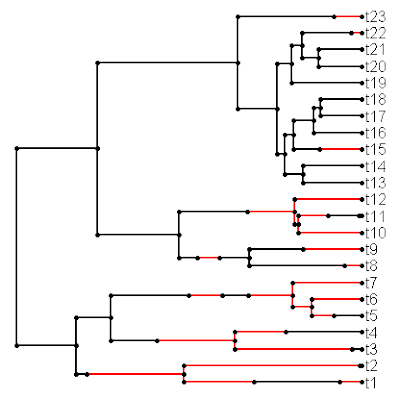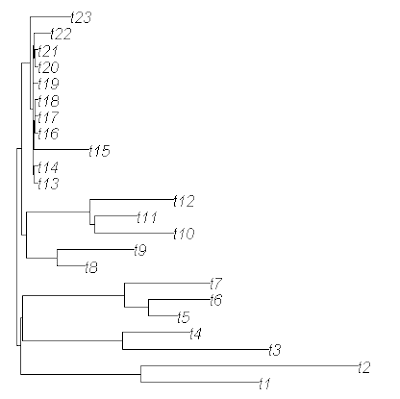Friday, September 23, 2011

Simulating multiple evolutionary rates on a tree

I just created a function that will simulate multiple evolutionary rates in different parts of a phylogeny with branch lengths. The different rate regimes are specified by the user using the stochastic mapping format of make.simmap(), read.simmap(), and the new function sim.history(). It is available from my R phylogenetics page (direct link to code here) and will eventually be part of the "phytools" package.

The way this function works is incredibly simple. It essentially acts as an elaborate wrapper for my fast Brownian motion simulator, fastBM(). Basically, it creates a new "phylo" object in which the parts of each branch in each rate are scaled by the rate. This was straightforward to do from the command like with simmap format trees before. Say, for instance, we had two mapped states "blue" and "green"; and we wanted to simulate a rate of σ2=1.0 on the "blue" branches and a rate of σ2=10 on the "green" branches, we could just do the following:

> tree<-mtree
> tree\$edge.length<-mtree\$mapped.edge[,"blue"]*1.0+ mtree\$mapped.edge[,"green"]*10
> x<-fastBM(tree)

The reason this works is because the realized outcome from a stochastic simulation on a tree (that is, the variance among species) is a function of the elapsed time (the branch length) multiplied by the evolutionary rate or instantaneous variance of the BM process on that branch. By first scaling each branch or branch segment by the desired evolutionary rate, we can then evolve by BM with a rate of σ2=1.0 on the rescaled tree to obtain the desired evolutionary rate heterogeneity.

The new function sim.rates() exploits the same principle. Let me illustrate sim.rates() with an example that also uses sim.history().

First, load "phytools" and the source files for sim.rates() and sim.history():

> require(phytools)
> source("sim.history.R")
> source("sim.rates.R")

Now, let's simulate a stochastic tree and character history using the "ape" function rbdtree() and sim.history(). We can also plot the history using plotSimmap():

> tree<-rbdtree(b=1,d=0,Tmax=log(12.5))
> Q<-matrix(c(-1,1,1,-1),2,2) # for sim.history
> mtree<-sim.history(tree,Q)
> plotSimmap(mtree)

Now, we should have something that looks like this:Next, let's simulate on this tree using two different rates: σ2=1 on the branches and branch segments labeled "1" (and painted black in the figure above); and σ2=20 along the branches labeled "2" (red branches). If we turn the option plot to TRUE, we can even visualize the rate heterogeneity:

> rates<-c(1,20); names(rates)<-c(1,2)
> x<-sim.rates(mtree,sig2=rates,plot=T)Finally, if we'd like to "prove" to ourselves that the data in x has indeed been generated under the intended two-rate model, we can fit the model to our tree & data using brownie.lite() (which is based on O'Meara et al. 2006):

> brownie.lite(mtree,x)
. . .
\$sig2.multiple
1 2
0.7987023 22.5603855
\$a.multiple
 1.47557
\$vcv.multiple
1 2
1 0.1720410 -0.5169118
2 -0.5169118 81.5076461
\$logL.multiple
 -50.03446
\$k2
 3
\$P.chisq
 0.0005468257
\$convergence
 "Optimization has converged."

(Some parts of the output from brownie.lite() have been excluded here).

Cool!

1.All I have to say is: cool!

2.wow Liam, thank you so much for putting this out. The timing couldn't have been more perfect for me, as I was trying to figure out how to get around creating a null distribution of traits in a morphospace under multiple rates BM, to calculate Sidlauskas' (2008) statistics for para/polyphyletic groups. I'll give it a try with your functions readily! Thanks again!

3.Hi Liam, I'm wondering how could I create a mapped tree with each branch (edge) being a diffferent state? and therefore be able to provide a vector of rates equal to the # branches. Thanks in advance, Martha

Note: due to the very large amount of spam, all comments are now automatically submitted for moderation.